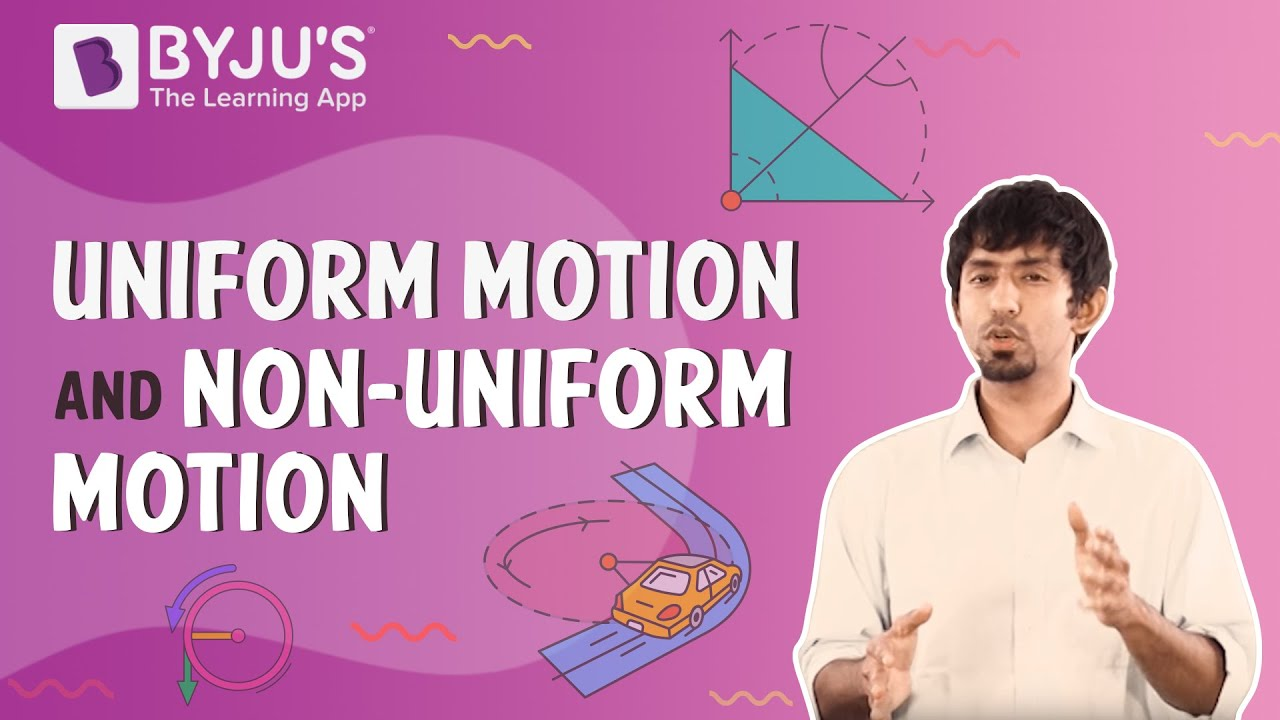# What is uniform motion and non-uniform motion? Give examples.

Based on the distance travelled and the amount of time spent, there are two kinds of motion which are uniform and non-uniform motion.

## Uniform motion

Uniform motion is defined as the motion of an object on a straight line with constant velocity along that line, regardless of time, because it occupies equal distances at equal time intervals.

Examples:

1. The motion of the earth around the sun
2. In swing machine, vibrating spring
3. Movement of the ceiling fan’s blades.
4. If a car’s speed is 10 m/s, it implies that in one second the car covers 10 meters. The speed is unchanged in each second.
5. Pendulum with equivalent amplitude on either side

## Non-uniform motion

Non-uniform motion is defined as the movement of an object at varying speeds and does not cover the same distance at equal time intervals, regardless of the time interval’s duration.

Examples:

1. Moving train
2. If in the first two seconds, a car occupies 10 meters and in the next two seconds it covers15 meters.
3. Bouncing ball
4. Asteroid movement
5. Movement of an aeroplane in clouds and landing
6. Two cars colliding each other(10)(5)